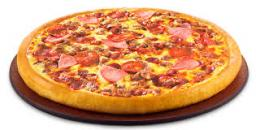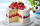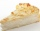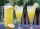# Pizza 5

You have 2/4 of a pizza, and you want to share it equally between 2 people. How much pizza does each person get?

x =  0.25

### Step-by-step explanation:

$x=\frac{2}{4}\mathrm{/}2=\frac{1}{4}=0.25$Did you find an error or inaccuracy? Feel free to write us. Thank you!Tips to related online calculators
Need help to calculate sum, simplify or multiply fractions? Try our fraction calculator.

## Related math problems and questions:

• Barbara 2Barbara get 6 pizzas to divide equally among 4 people. How much of a pizza can each person have?
• Equivalent expressionsA coach took his team out for pizza after their last game. There were 14 players, so they had to sit in smaller groups at different tables. Six players sat at one table and got 4 small pizzas to share equally. The other players sat at the different table
• Four peopleFour people want waffles for breakfast. There are 6 waffles left. How can 6 waffles be shared equally among 4 people? How much does each person get? Draw a picture and write a division expression to model the problem.
• Julian 2Julian and two of his friends are going to share 1/4 of a pizza. How much will each person get?
• Pizza palaceJosh is at Enzo's pizza palace. He can sit at a table with 5 of his friends or at a different table with seven of his friends. The same size pizza is shared equally among the people at each table. At which table should Josh sit to get more pizza? (write t
• JerryJerry has 3/4 of a pizza. He needs to share it with 6 friends. What fraction of the pizza will each friend get? Only write the fraction
• Cake 71/3 of a cake shared with 4 people. What share of the whole cake has each people?
• Mixed divided with fracThere are 2 2/3 pizzas. How many people are sharing when each has 2/3 of pizza?
• Pizza fractionsAnn ate a third of a pizza and then another quater. Total part of pizza eaten by Ann and how much pizza is left?
• One fourthOne fourth of an apple pie is left for 2 family members to share equally. What fraction of the original pie will each member​ get?Six friends are sharing 8 cups of lemonade. If they share the lemonade equally, how many cups will each friend get?A pizza is cut into slices that are each 1/6 of the whole. John is going to eat 1/2 of the whole pizza. How many slices will John eat?5/8 of the pizza is covered in mushrooms, 1/4 of the pizza is covered with pineapples, and the rest of the pizza is covered with only cheese. What fraction of the pizza is covered with only cheese?Issac eats 1/6 of the pizza. Maya then eats 3/5 of the remaining pizza. What fraction of the original pizza is left?Five people have $122000 each, and one person has$539000. How much should each person (equally) pay?Marcus ate half pizza on monday night. He than ate one third of the remaining pizza on Tuesday. Which of the following expressions show how much pizza marcus ate in total?Maria ate 1/4 of a pizza. If there were 20 slices of pizza, how many slices did Maria eat?